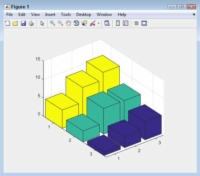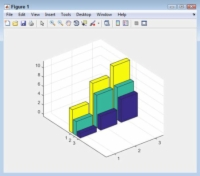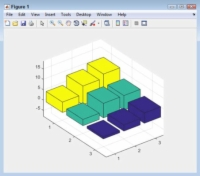How to Modify the Aspect Ratio to Create a 3D Effect for Your MATLAB Plots - dummies

How to Modify the Aspect Ratio to Create a 3D Effect for Your MATLAB Plots

How the 3D effect appears onscreen depends on the data aspect ratio you set in MATLAB. The daspect() function lets you obtain the current aspect ratio and set a new one. The aspect ratio is a measure of how the x-, y-, and z-axis interact.

For example, an aspect ratio of [1, 2, 3] would mean that for every 1 unit of the x-axis, there are two units of the y-axis and three units of the z-axis. Perform the following steps to see how this feature works:

1Type YSource = [1, 3, 5; 3, 7, 9; 5, 7, 11]; and press Enter.

MATLAB creates a data source for you.

2Type Bar1 = bar3(YSource); and press Enter.

You see a 3D bar chart appear.3Type rotate(Bar1, [0, 0, 1], 270); and press Enter.

The bar chart rotates so that you can see the individual bars easier.

4Type daspect() and press Enter.

The output contains three values, like this:

ans =
0.3571 0.2679 2.1670

So, you now know the current aspect ratio of the plot, with the first number representing the x-axis value, the second number the y-axis value, and the third number the z-axis value.5Type daspect([.25, 1, 1.2]); and press Enter.

The data aspect ratio changes to create tall, skinny-looking bars. The data is precisely the same as before, as is the rotation, but the interpretation of the data changes.6Type daspect([.65, .5, 7]); and press Enter.

The impression is now that the differences between the data points are actually quite small. Again, nothing has changed in the data or the rotation. The only thing that has changed is how the data is presented.

7Type daspect(‘auto’) and press Enter.

The data aspect returns to its original state.